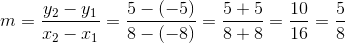# ISEE Middle Level Math : How to find a line on a coordinate plane

## Example Questions

### Example Question #1 : How To Find A Line On A Coordinate Plane

Give the slope of the line that passes through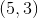and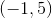.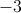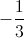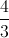Explanation:

Use the slope formula, substituting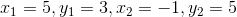: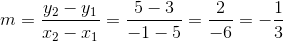### Example Question #1 : How To Find A Line On A Coordinate Plane

What is the slope of the line that passes through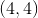and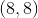?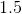Explanation:

We can use the slope formula: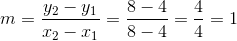### Example Question #2 : How To Find A Line On A Coordinate Plane

What is the slope of the line that passes through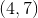and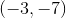?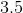Explanation:

We can use the slope formula: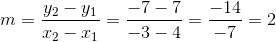### Example Question #11 : Coordinate Geometry

Find the slope of the line that passes through coordinates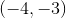and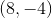.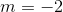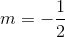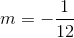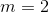Explanation:

The formula for slope is: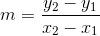In this particular question our values are given as follows.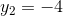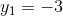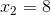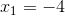Substituting the above values into the formula for slope we get,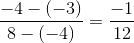.

### Example Question #3 : How To Find A Line On A Coordinate Plane

Billy set up a ramp for his toy cars. He did this by taking a wooden plank and putting one end on top of a brick that was 3 inches high. He then put the other end on top of a box that was 9 inches high. The bricks were 18 inches apart. What is the slope of the plank?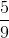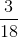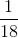Explanation:

The value of the slope (m) is rise over run, and can be calculated with the formula below:The coordinates of the first end of the plank would be (0,3), given that this is the starting point of the plank (so x would be 0), and y would be 3 since the brick is 3 inches tall.

The coordinates of the second end of the plank would be (18,9) since the plank is 18 inches long (so x would be 18) and y would be 9 since the box was 9 inches tall at the other end.

From this information we know that we can assign the following coordinates for the equation: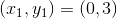and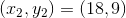Below is the solution we would get from plugging this information into the equation for slope: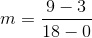This reduces to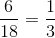### Example Question #1 : How To Graph A Line

What is the slope of the line depicted by the graph?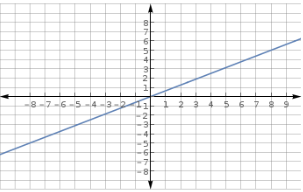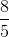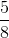Explanation:

Looking at the graph, it is seen that the line passes through the points (-8,-5) and (8,5).The slope of a line through the points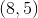and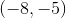can be found by setting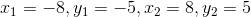:

in the slope formula: